Technical Information Site of Power Supply Design

• Basic Knowledge
• DC/DC
• Parallel Connections of LDO Linear Regulators
• Parallel Connection of LDOs Using Ballast Resistors

2021.01.13 DC/DC

# Parallel Connection of LDOs Using Ballast Resistors

Parallel Connections of LDO Linear Regulators

In the previous article, we explained parallel connection using diodes as one of two methods for parallel connection of LDOs. This article concerns the other method, parallel connection using ballast resistors.

Parallel Connection of LDOs Using Ballast Resistors

An example of parallel connection using resistors is shown. Because resistors are inserted in series in the output path, as the load current increases, the output voltage falls.

In this circuit also, current begins to be supplied from the LDO with the higher output voltage. The output current of the LDO for which the voltage is higher flows through a ballast resistor so that a voltage drop occurs, and when the voltage becomes the same as the lower LDO voltage, current begins to be supplied from the LDO with the lower voltage as well. In this way, voltage drops across resistors are utilized to balance the output voltages while supplying currents from each of the LDOs to the load. The relation according to which the output voltages are balanced can be represented by the following equation.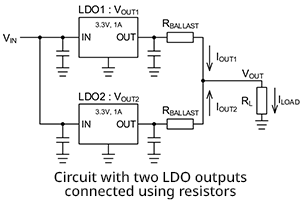VOUT1-IOUT1×RBALLAST=VOUT2-IOUT2×RBALLAST

VOUT1：LDO1 output voltage
VOUT2：LDO2 output voltage
IOUT1：LDO1 output current
IOUT2：LDO2 output current
RBALLAST：Ballast resistance

The relationship between the ballast resistance, the current divided between IOUT1 and IOUT2, and the voltage difference between the LDOs, is expressed by the following equation.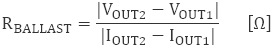From this equation, it is seen that if an attempt is made to improve the balance between the currents IOUT1 and IOUT2, the resistance value of the ballast resistors RBALLAST becomes high, and even when the output voltage difference between the LDOs is large, RBALLAST is high. When the resistance value is high, the voltage drop at the load point increases.

For example, if the deviation at 3.3 V and 1 A output is +1% for LDO1 and -1% for LDO2, the output voltage difference is 66 mV (2% of 3.3 V). If the load current of 2 A is to be divided into 1.2 A and 0.8 A currents, from the above equation, the ballast resistance will be 0.165 Ω.

The graph on the right indicates how the output currents from the LDOs are apportioned and supplied to the load. Up until a load current of 0.4 A, only current from LDO1 flows because the output voltage of LDO1 is higher. From about 0.4 A, the voltage drop due to the ballast resistors exceeds 66 mV (= 0.4 A × 0.165 Ω), and so the output voltages of LDO1 and LDO2 become the same at the VOUT points, so that current from LDO2 begins to flow. When at 2 A, the currents supplied are 1.2 A and 0.8 A, just as calculated.

The goal is to parallel-connect two LDOs each with a 1 A output to obtain a 2 A output, but using LDOs for which the maximum recommended output current is 1 A, when one of the LDOs has reached 1 A, the maximum output current for the system has been reached. Hence, referring to the graph, the maximum output current that can be supplied is only 1.6 A.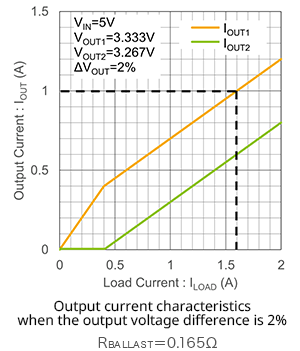The graph shown next is a load regulation graph. As the load current increases, the voltage drops due to the ballast resistors grow larger. The voltage drops can be calculated using the following equation. For actual voltage drops, this voltage is added to the load regulation voltages of the LDOs themselves.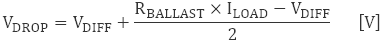VDIFF：Difference in output voltages of LDO1 and
LDO2
RBALLAST：Ballast resistance
* Region in which output currents from both LDO1 and
LDO2 are flowing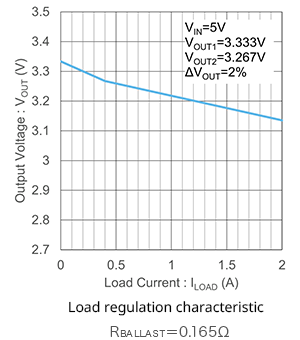Next, an example for LDOs with an output voltage tolerance of ±3%, including the temperature characteristic, is shown. When, for an output of 3.3 V and 1 A, the deviation is +3% for LDO1 and -3% for LDO2, the output voltage difference is 198 mV (6% of 3.3 V). When the load current of 2 A is apportioned as 1.2 A and 0.8 A, the ballast resistance is 0.495 Ω.

The distribution of output currents from the LDOs that are supplied to the load are shown in the graph on the lower left. Similarly to the example above in which there is a ±1% variation in the output voltages, the currents are distributed according to calculations. The graph on the lower right shows the load regulation under these conditions. To the extent that the ballast resistance value is increased, the voltage drop due to the load current is larger.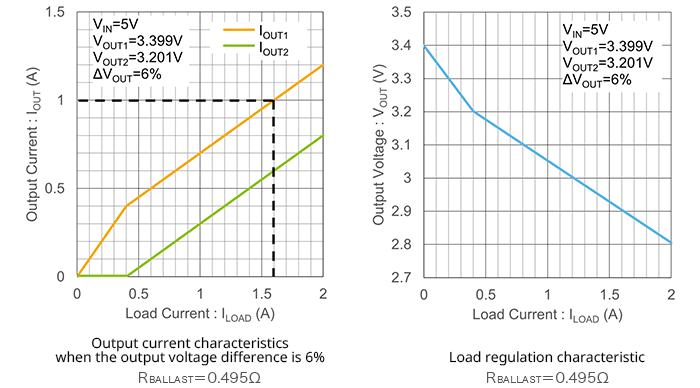As we have explained, there is a tradeoff between the output voltage difference of the LDOs and the distribution of the output currents on the one hand, and the output voltage drop on the other. The ballast resistance value must be chosen considering the balance between all relevant characteristics. Since output currents flow through the ballast resistors, large power losses occur. It is important to confirm that the rated power for the resistors used satisfies specifications. JEITA (in its Guidelines of notabilia for fixed resistors for use in electronic equipment, RCR-2121A/B) recommends that resistors be used at or below 50% of the rated power.

## Key Points

・Parallel connections using ballast resistors can cause output currents to be distributed according to a value calculated using an equation.

・Output currents flow in the ballast resistors, so that a voltage drop occurs at the load point, and power losses occur in the resistors.

・There is a tradeoff between the difference in the LDO output voltages, the output current distribution, and the output voltage drop. The value of the ballast resistors must be determined while balancing all characteristics.